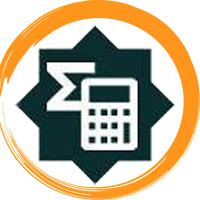# Free Book Apps

Free book apps for Android

Showing posts with label Learn Java.math package. Show all posts

## Learn Java.math packageLearn Java.math package
Java.math package provides classes for performing arbitrary-precision integer arithmetic (BigInteger) and arbitrary-precision decimal arithmetic (BigDecimal). This reference will take you through simple and practical methods available in java.math package.
This reference has been prepared for the beginners to help them understand the basic functionality related to all the methods available in Java.math package.
Before you start doing practice with various types of examples given in this reference, I'm making an assumption that you are already aware of basic Java Programming.

Java.math package provides classes for performing arbitrary-precision integer arithmetic (BigInteger) and arbitrary-precision decimal arithmetic (BigDecimal). This reference will take you through simple and practical methods available in java.math package.Learn Java.math package